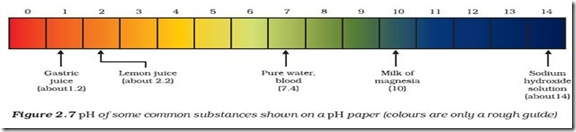# Chemistry X | Acids, Bases and Salts | HOW STRONG ARE ACID OR BASE SOLUTIONS?

HOW STRONG ARE ACID OR BASE SOLUTIONS?

We know how acid-base indicators can be used to distinguish between an acid and a base. We have also learnt in the previous section about dilution and decrease in concentration of H+ or OH– ions in solutions. Can we quantitatively find the amount of these ions present in a solution? Can we judge how strong a given acid or base is?

We can do this by making use of a universal indicator, which is a mixture of several indicators. The universal indicator shows different colours at different concentrations of hydrogen ions in a solution.

A scale for measuring hydrogen ion concentration in a solution, called pH scale has been developed. The p in pH stands for ‘potenz’ in German, meaning power. On the pH scale we can measure pH from 0 (very acidic) to 14 (very alkaline). pH should be thought of simply as a number which indicates the acidic or basic nature of a solution. Higher the hydronium ion concentration, lower is the pH value.

The pH of a neutral solution is 7. Values less than 7 on the pH scale represent an acidic solution. As the pH value increases from 7 to 14, it represents an increase in OH– ion concentration in the solution, that is,

increase in the strength of alkali. Generally paper impregnated with the universal indicator is used for measuring pH. One such paper is shown in Fig. 2.6.Activity 2.11

1. Test the pH values of solutions given in Table 2.2.
2. Record your observations.
3. What is the nature of each substance on the basis of your observations?The strength of acids and bases depends on the number of H+ ions and OH– ions produced, respectively. If we take hydrochloric acid and acetic acid of the same concentration, say one molar, then these produce different amounts of hydrogen ions. Acids that give rise to more H+ ions are said to be strong acids, and acids that give less H+ ions are said to be weak acids. Can you now say what weak and strong bases are?

## One comment on “Chemistry X | Acids, Bases and Salts | HOW STRONG ARE ACID OR BASE SOLUTIONS?”

This site uses Akismet to reduce spam. Learn how your comment data is processed.